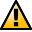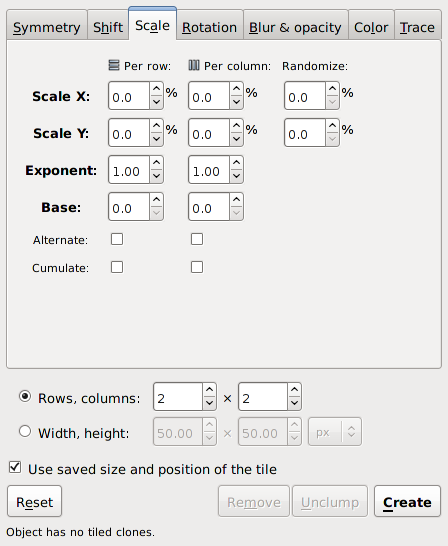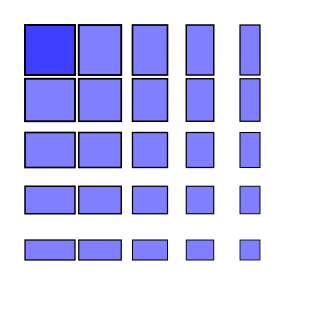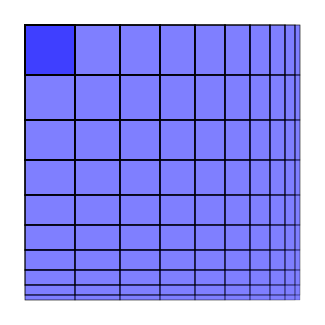WarningThis section not yet updated for Inkscape v0.92.

## Scale Tab

The Scale tab allows one to increase or decrease the size of the tiles depending on the row and column position. The following options are available to scale tiles:

• Scale X, Scale Y: Scales each tile in terms of percentage. A random factor can be added.
• Exponent: Scale each tile with an exponential factor. The nominal scaling S becomes Sexponent.
• Base: Used to create a logarithmic spiral along with Rotation. The nominal scaling S becomes baseS-1 unless base is one in which case scaling remains unchanged. Use a value less than 1 for a converging spiral and a value greater than 1 for a diverging spiral. A true logarithmic spiral would use a base of e = 2.718 (or 1/e = 0.368). See the Tile Tricks section for examples.
• Alternate: Alternate scaling up and scaling down tiles.
• Cumulate: Scaling is cumulative.The Scale tab of the Tile Clones dialog.A P1 symmetry tiling with a negative scaling. There is an −15% x scaling for each column and a −15% y scaling for each row. The scaling is a percentage of the base tile bounding box. The spacing between the center of adjacent tiles remains fixed.A P1 symmetry tiling with a cumulative negative scaling. There is a −10% x scaling for each column and a −10% y scaling for each row. There is also a −5% x shift for each column and a −5% y shift for each row. The Cumulate box is checked for both x and y. A general rule is that to keep scaled tiles just touching, specify a cumulative shift that is half of the scaling (in percent).